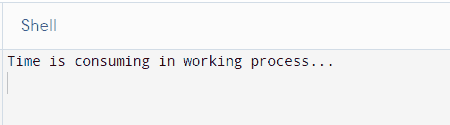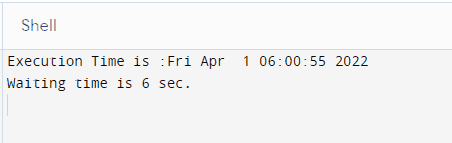# The time Module in Python

Rana Hasnain Khan Apr 12, 2022

We will introduce the Python `time` module and its functions in Python with examples. We will also introduce different methods that can be used related to time in Python with examples.

## Python Time Module

Python’s `time` module provides a library for reading, representing, and resetting time data in various ways. In Python, date, time, and date-time are all objects, so we’re manipulating objects rather than strings or timestamps whenever we manipulate them.

We’ll talk about the `time` module in this tutorial, which allows us to handle numerous tasks in real-time. The time module belongs to the EPOCH convention, which denotes the beginning of time.

Time on the Unix system EPOCH began on January 1, 1970, at 12:00 a.m. and ended on December 31, 2038.

Type the following code to determine the EPOCH time value on your machine. Importing time is essential; the program cannot work without it, as shown below.

``````# python
import time

print(time.gmtime(0))
``````

Output:As you can see, it displays the time of the beginning of the EPOCH.

Now, let’s discuss what is a `tick` in Python.

### `tick` in Python

A `tick` is a floating-point value measured in seconds, representing a time interval. We have Daylight Saving Time (DST) when the clock changes one hour forward in the summer and back in the fall.

The time function module in Python has many time functions that we utilize in our programming activities. When working on different Python platforms, different scenarios arise that necessitate the use of specific time functions.

Now, let’s discuss some functions that our time module provides with easy-to-use examples that will help us understand the usage and advantages of these functions in our python applications.

### `time.time()` Function in Python

The core function of the `time` module is `time()`. As a floating-point value, it counts the number of seconds since the epoch (1970).

Syntax:

``````# python
import time
print("Seconds passed since the epoch are : ", time.time())
``````

Output:As you can see from the above example, it returned the total number of seconds from 1970.

Now, let’s imagine if we want to find the number of seconds it took to complete a particular process.

The time function is also used to find the time in seconds between the two points. So, let’s go through an example in which we will add the waiting time using the `time.sleep()` function in seconds, as shown below.

``````# python
import time

Start= time.time()

print("Time is consuming in working process...")

time.sleep(6)

End = time.time()

print("Time consumed in working process: ",End - Start)
``````

Output:As you can see from the above example, we can easily get the time consumed for any process using starting and ending times. Now, let’s discuss another function that is `time.ctime()` in detail.

### `time.ctime()` Function in Python

The `time.time()` method accepts an input of “seconds since the epoch” and converts it to a human-readable string based on the current time. It provides the present time if no parameter is supplied.

When we need to find the time after a given time, this program will instruct us on how to do so. This software will tell you the time after a set time has passed.

``````# python
import time

print('The present time is :', time.ctime())

Updated = time.time() + 120

print('After 60 secs from present time :', time.ctime(Updated))
``````

Output:As you can see from the above example, we can quickly get the future time by giving `ctime()` the number of seconds for which we want the time.

We will now discuss another useful function, `time.sleep()`.

### `time.sleep()` Function in Python

The `time.sleep()` function pauses the current thread’s execution for seconds. To acquire a more accurate sleep time, use a floating number of points as input.

The `sleep()` function can be applied when we wait for a file to finish or the data to publish.

Now, let’s go through an example in which the `ctime()` function is used to present the current time, and then the `sleep()` function is used to pause the execution for a specific time.

``````# python
import time

print (" Execution Time is :",end="")

print (time.ctime())

print ('Waiting time is 6 sec.')

time.sleep(6)

print (" Exicution Time ends at : ",end="")

print (time.ctime())
``````

Output:As you can see from the above example, using the `time.sleep()` function, we can make a process wait for as many seconds as we want. As shown in the above results, the function will work fine after the wait is over.

Now we will discuss a class `time.struct_time` in `time` module in detail.

### `time.struct_time` Class in Python

The single data structure is `time.struct_time` in the `time` module. It features a named tuple structure and can be obtained via indexes or attribute values.

1. This class is useful when you need to access a specific date.
2. `Localtime()`, `gmtime()`, and return the `struct_time` objects are among the functions provided by this class

Let’s go through an example and check how we can use it in our applications.

``````# python
import time

print('Present local time:', time.ctime())

time = time.localtime()

print('Days according to monthly:', time.tm_mday)

print('Days according to week :', time.tm_wday)

print('Days according to year :', time.tm_yday)
``````

Output:As you can see from the above example, we can quickly get days according to month, week, and year using different methods. Now, we will discuss another useful function, `time.strftime()` provided by the `time` module in Python.

### `time.strftime()` Function in Python

The second argument to this method is a tuple or struct time, which transforms into a string using the format provided over the first parameter.

``````# python
import time

PRESENT = time.localtime(time.time())

print("Current date time is: ",time.asctime(PRESENT))

print(time.strftime("%y/%m/%d %H:%M", PRESENT))

print(time.strftime("%a %b %d", PRESENT))

print(time.strftime("%c", PRESENT))

print(time.strftime("%I %p", PRESENT))

print(time.strftime("%Y-%m-%d %H:%M:%S %Z", PRESENT))
``````

Output:As you can see from the above example, we can get different time formats using this function. In this tutorial, we discussed many valuable functions in the time module.

Rana is a computer science graduate passionate about helping people to build and diagnose scalable web application problems and problems developers face across the full-stack.